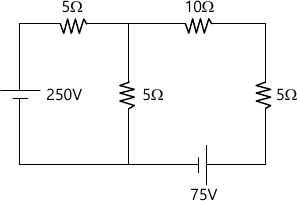MORE IN Basic Electrical Engineering (BEE)
SPPU First Year Engineering (Semester 2)
Basic Electrical Engineering (BEE)
April 2013
Total marks: --
Total time: --
INSTRUCTIONS
(1) Assume appropriate data and state your reasons
(2) Marks are given to the right of every question
(3) Draw neat diagrams wherever necessary

Answer any one question from Q1 & Q2
1 (a) What is the insulation resistance? Derive an expression for the Insulation resistance of a single core cable.
6 M
1 (b) Derive an expression for energy stored per unit volume in the magnetic field.
6 M

2 (a) An iron ring has a mean circumference of 180 cm. It carries a current Of 1.5 Amp and has 600 turns of coil wound over it. The relative Permeability of the iron is 1200. Calculate 1) MMF 2) Field strength and 3) flux density.
6 M
2 (b) A piece of silver wire has a resistance of 3?. What will be the resistance of a manganin wire one-third the length and one-third the diameter that of silver? The resistivity of manganin is 30 times that of silver.
6 M

Answer any one question from Q3 & Q4
3 (a) Derive an expression of R.M.S. value of an sinnsoidally varying Currrent.
6 M
3 (b) Derive an expression of EMF induced in a single phase transformer.
6 M

4 (a) An 80KVA, 3200/400v, 50Hz, single phase transformer has III turns On the secondary calculate 1) No of turns on primary 2) secondary full load current 3) c/s area of the core if the maximum flux density is 1.2 tesla.
6 M
4 (b) A 50?F capacitor is connected across a single phase 230v, 50Hz Supply. Calculate 1) the reactance offered by the capacitor 2) the Maximum current an d 3) the rms value of the current drawn by the Capacitor.
6 M

Answer any one question from Q5 & Q6
5 (a) the voltage and current, in simple series circuit are given by v=150 ? 30° and i=2?-15° if the supply frequency is 50Hz, determine, impedance, resistance, reactance and power consumed by the circuit.
6 M
5 (b) Derive the relation of line & phase values of voltage and current for three phase delta connected balanced load, with phasor.
7 M

6 (a) Sketch and explain the phasor diagrams of R-L-C series CKT. When 1) XL >XC and 2) XL< XC
6 M
6 (b) Two circuits, the impedance of which are given by Z1=(10+j15)? and Z2=(6-j8)? are connected in parallel across on A.C. supply. If the total Current supplied is 15 Amp, what are the branch current drawn by two circuits.
7 M

Answer any one question from Q7 & Q8
7 (a) State and explain kirchhoff's laws.
5 M
7 (b) Apply thevenin's theorem to calculate current drawn by ohm Resistance for the circuit shown in fig. 18 M

8 (a) Calculate current flowing in 8 ohm resistance for the circuit shown in Fig.1 applying superposition theorem.8 M
8 (b) Derive formulae to convert DELTA connected network into its STAR connected equivalent network
5 M

More question papers from Basic Electrical Engineering (BEE)